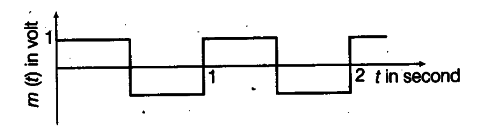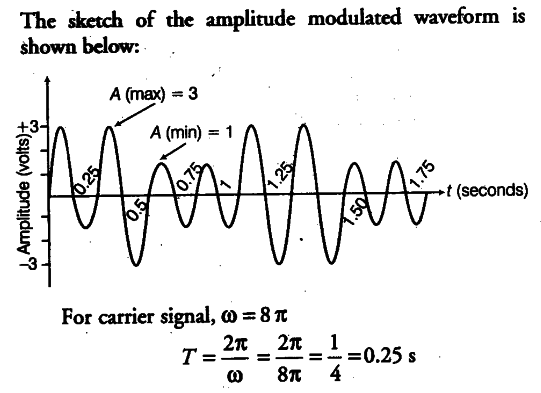# A modulating signal is a square wave as shown in the figure

A modulating signal is a square wave as shown in the figure.Sketch the amplitude modulated waveform.

Given, the equation of carrier wave,
c® = 2sin(8$\pi$t)
(i) According to the figure,
Amplitude of modulating signal,
{ A }_{m } = 1V
Amplitude of carrier wave,

{ A }_{c } = 2

{ T }_{m } = 1s